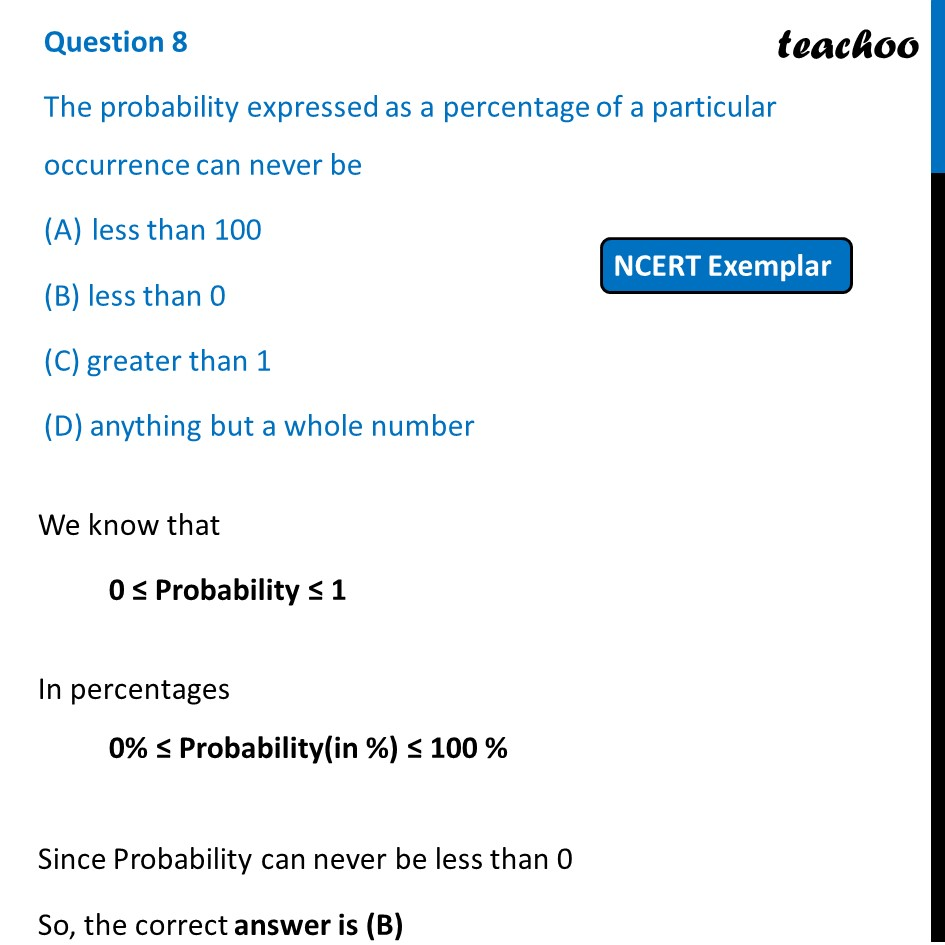NCERT Exemplar - MCQ

Chapter 14 Class 10 Probability
Serial order wise

## (D) anything but a whole numberLearn in your speed, with individual attention - Teachoo Maths 1-on-1 Class

### Transcript

Question 8 The probability expressed as a percentage of a particular occurrence can never be less than 100 (B) less than 0 (C) greater than 1 (D) anything but a whole number We know that 0 ≤ Probability ≤ 1 In percentages 0% ≤ Probability(in %) ≤ 100 % Since Probability can never be less than 0 So, the correct answer is (B)